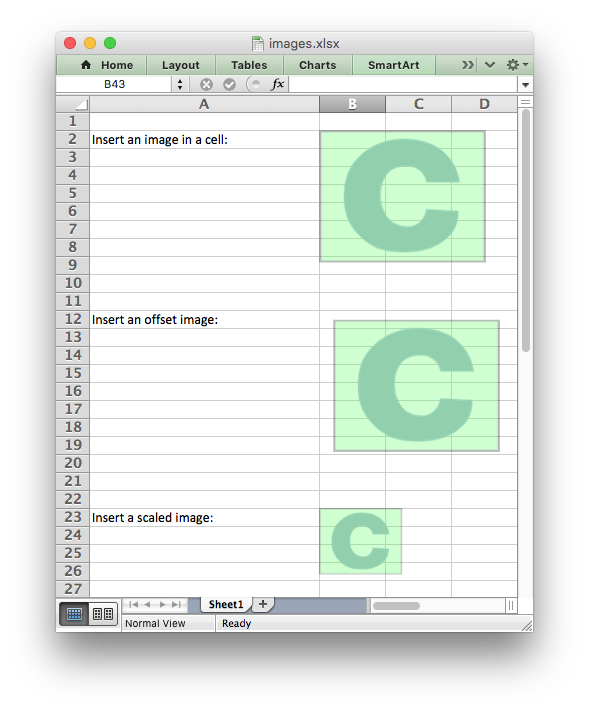libxlsxwriter
images.c

Example of adding images to a worksheet./*
* An example of inserting images into a worksheet using the libxlsxwriter
* library.
*
* Copyright 2014-2021, John McNamara, jmcnamara@cpan.org
*
*/
#include "xlsxwriter.h"
int main() {
/* Create a new workbook and add a worksheet. */
lxw_workbook *workbook = workbook_new("images.xlsx");
lxw_worksheet *worksheet = workbook_add_worksheet(workbook, NULL);
/* Change some of the column widths for clarity. */
worksheet_set_column(worksheet, COLS("A:A"), 30, NULL);
/* Insert an image. */
worksheet_write_string(worksheet, CELL("A2"), "Insert an image in a cell:", NULL);
worksheet_insert_image(worksheet, CELL("B2"), "logo.png");
/* Insert an image offset in the cell. */
worksheet_write_string(worksheet, CELL("A12"), "Insert an offset image:", NULL);
lxw_image_options options1 = {.x_offset = 15, .y_offset = 10};
worksheet_insert_image_opt(worksheet, CELL("B12"), "logo.png", &options1);
/* Insert an image with scaling. */
worksheet_write_string(worksheet, CELL("A22"), "Insert a scaled image:", NULL);
lxw_image_options options2 = {.x_scale = 0.5, .y_scale = 0.5};
worksheet_insert_image_opt(worksheet, CELL("B22"), "logo.png", &options2);
/* Insert an image with a hyperlink. */
worksheet_write_string(worksheet, CELL("A32"), "Insert an image with a hyperlink:", NULL);
lxw_image_options options3 = {.url = "https://github.com/jmcnamara"};
worksheet_insert_image_opt(worksheet, CELL("B32"), "logo.png", &options3);
workbook_close(workbook);
return 0;
}
workbook_close
lxw_error workbook_close(lxw_workbook *workbook)
Close the Workbook object and write the XLSX file.
lxw_image_options::url
char * url
Definition: worksheet.h:1733
workbook_new
lxw_workbook * workbook_new(const char *filename)
Create a new workbook object.
worksheet_insert_image
lxw_error worksheet_insert_image(lxw_worksheet *worksheet, lxw_row_t row, lxw_col_t col, const char *filename)
Insert an image in a worksheet cell.
lxw_worksheet
Struct to represent an Excel worksheet.
Definition: worksheet.h:2107
COLS
#define COLS(cols)
Convert an Excel A:B column range into a (col1, col2) pair.
Definition: utility.h:63
lxw_image_options::x_offset
int32_t x_offset
Definition: worksheet.h:1704
lxw_image_options
Options for inserted images.
Definition: worksheet.h:1701
lxw_workbook
Struct to represent an Excel workbook.
Definition: workbook.h:280
worksheet_write_string
lxw_error worksheet_write_string(lxw_worksheet *worksheet, lxw_row_t row, lxw_col_t col, const char *string, lxw_format *format)
Write a string to a worksheet cell.
worksheet_insert_image_opt
lxw_error worksheet_insert_image_opt(lxw_worksheet *worksheet, lxw_row_t row, lxw_col_t col, const char *filename, lxw_image_options *options)
Insert an image in a worksheet cell, with options.
worksheet_set_column
lxw_error worksheet_set_column(lxw_worksheet *worksheet, lxw_col_t first_col, lxw_col_t last_col, double width, lxw_format *format)
Set the properties for one or more columns of cells.
CELL
#define CELL(cell)
Convert an Excel A1 cell string into a (row, col) pair.
Definition: utility.h:45